### Home > A2C > Chapter 9 > Lesson 9.2.3 > Problem9-112

9-112.
1. Kahlid is going to make a table to graph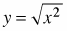, but Aaron says that it would be a waste of time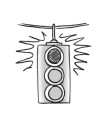to make a table because the graph is the same as y = x. Homework Help ✎

1. Use Kahlid's equation to make the table and draw the graph.

2. As you can see, Aaron was wrong, but you did get a graph that you have seen before. What other equation has the same graph? Explain why this is reasonable.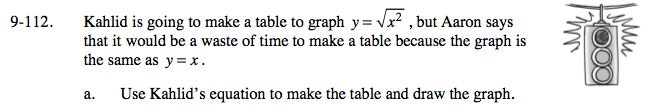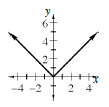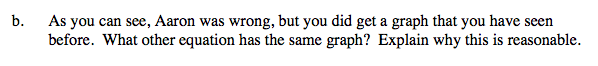y = |x|